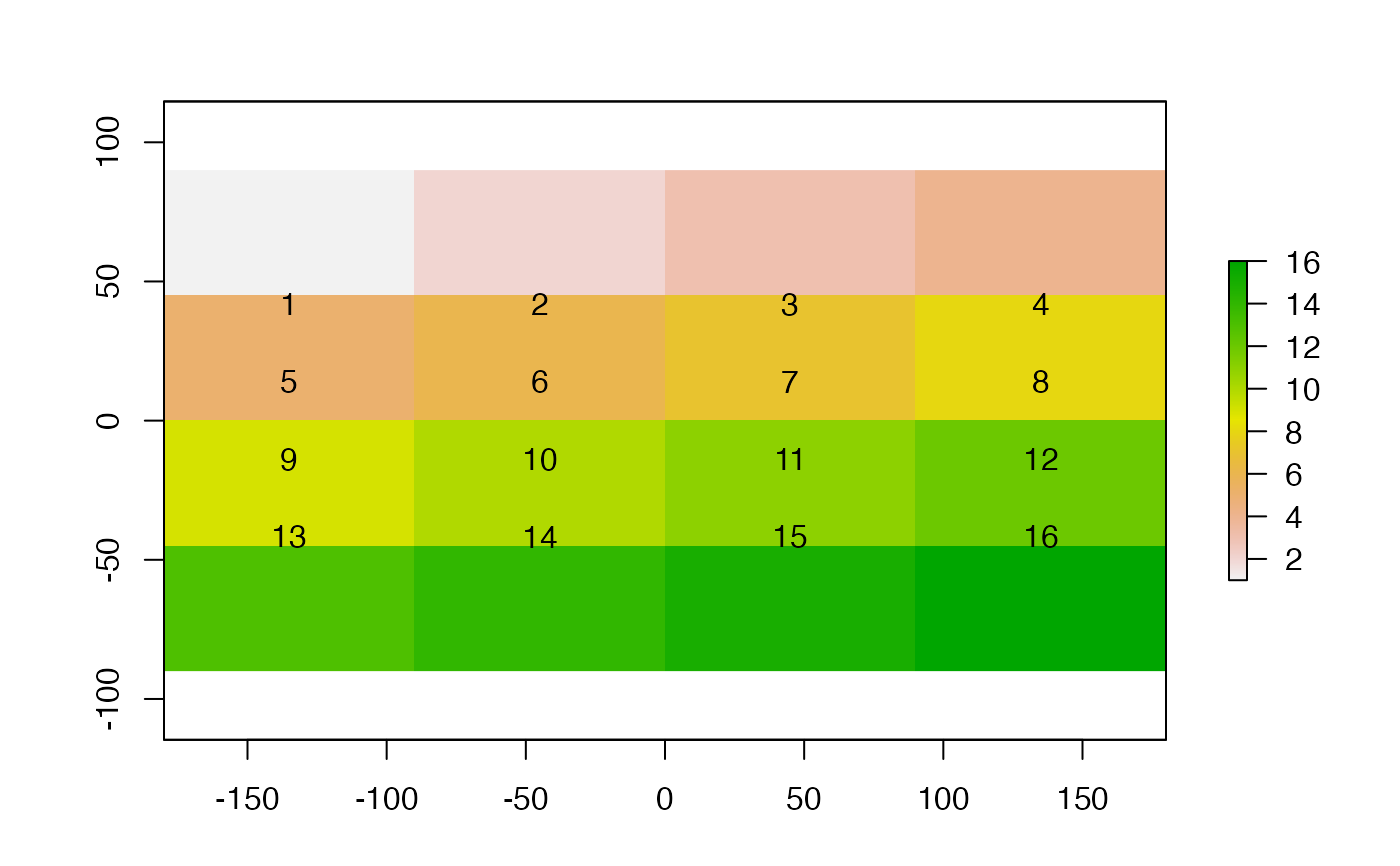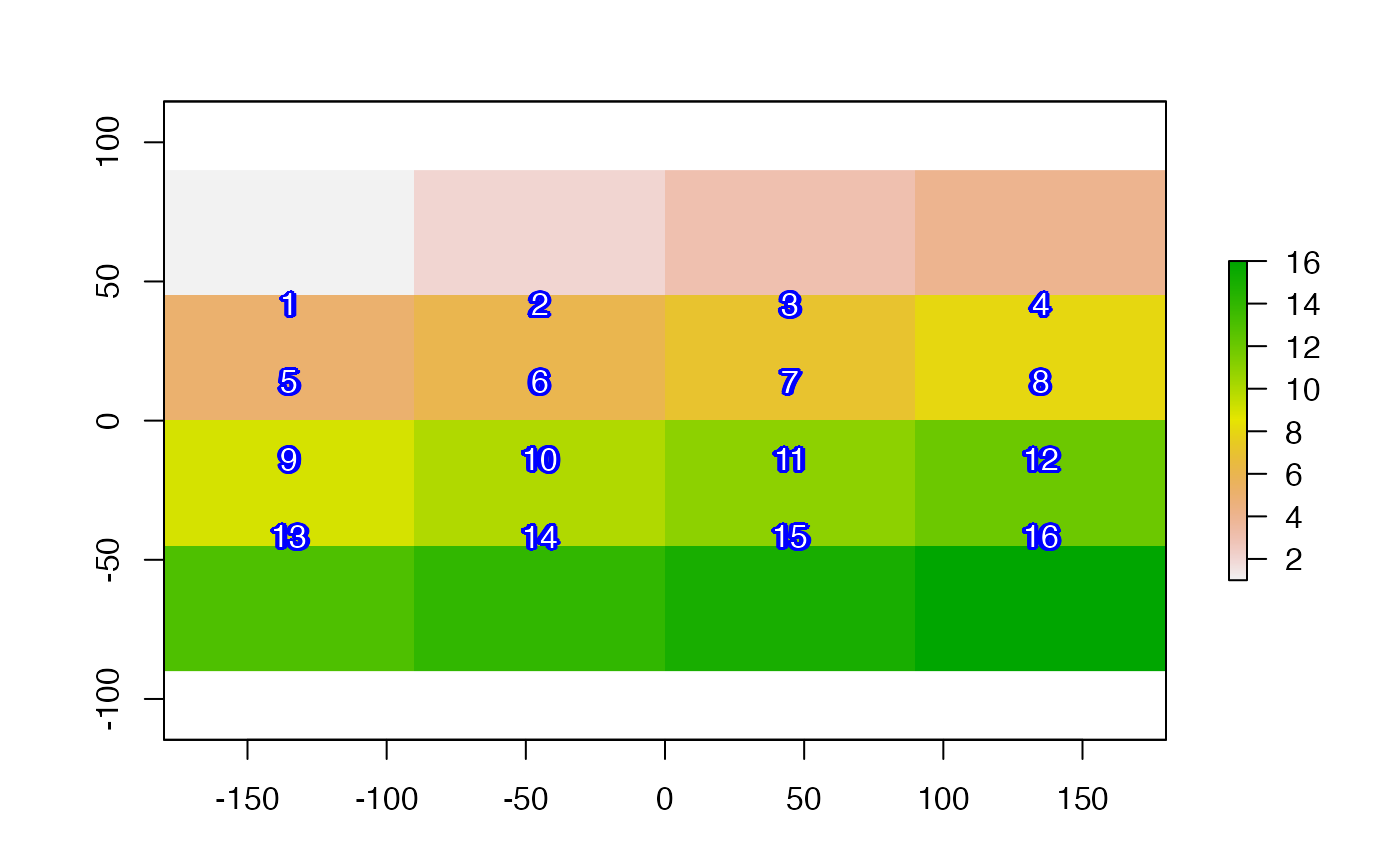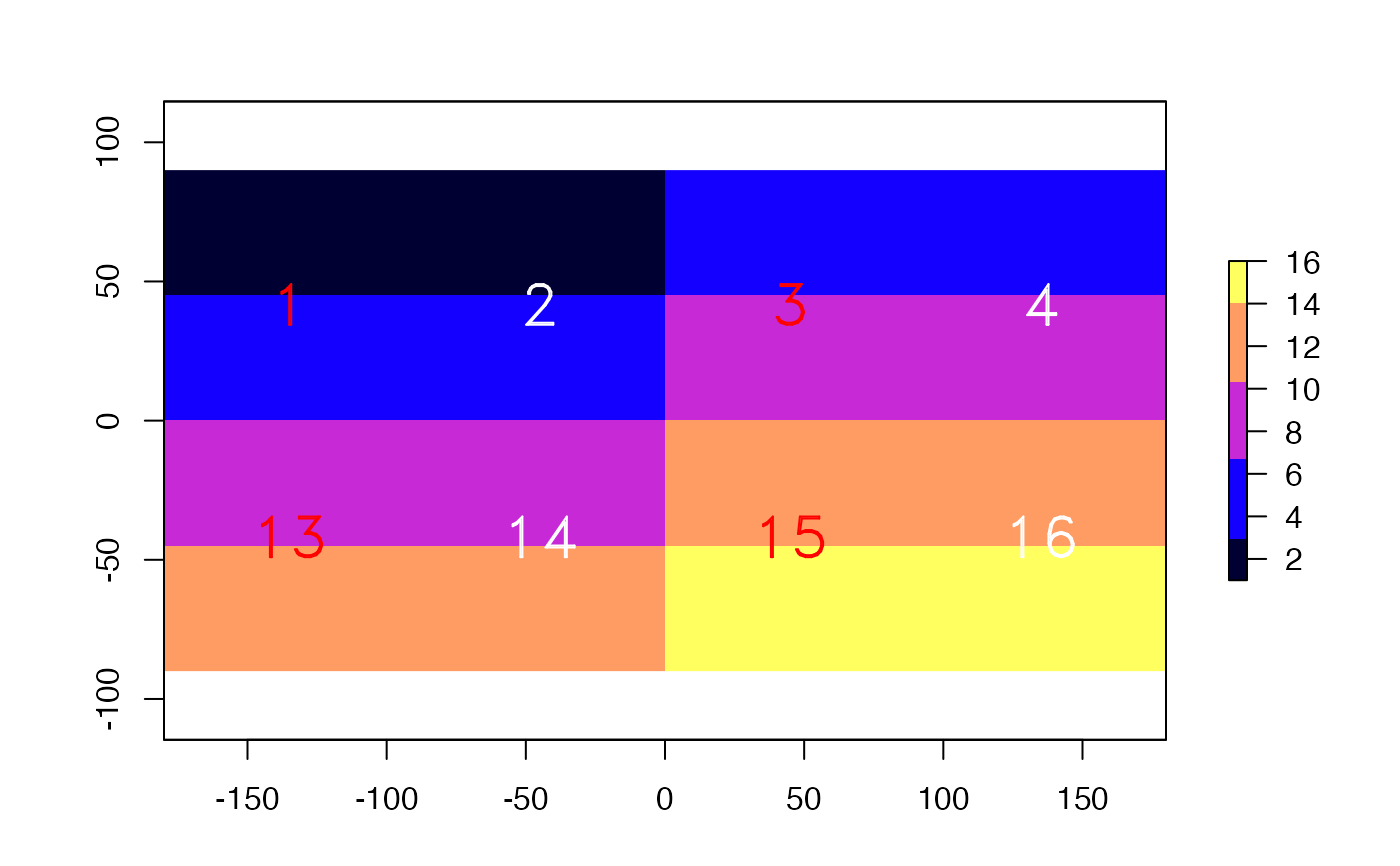Plots labels, that is a textual (rather than color) representation of values, on top an existing plot (map).

# S4 method for RasterLayer
text(x, labels, digits=0, fun=NULL, halo=FALSE, ...)

# S4 method for RasterStackBrick
text(x, labels, digits=0, fun=NULL, halo=FALSE, ...)

# S4 method for SpatialPolygons
text(x, labels, halo=FALSE, ...)

# S4 method for SpatialPoints
text(x, labels, halo=FALSE, ...)

## Arguments

x

Raster*, SpatialPoints* or SpatialPolygons* object

labels

character. Optional. Vector of labels with length(x) or a variable name from names(x)

digits

integer. how many digits should be used?

fun

function to subset the values plotted (as in rasterToPoints)

halo

logical. If TRUE a 'halo' is printed around the text. If TRUE, additional arguments hc='white' and hw=0.1 can be modified to set the colour and width of the halo

...

additional arguments to pass to graphics function text

text, plot

## Examples

r <- raster(nrows=4, ncols=4)
r <- setValues(r, 1:ncell(r))
plot(r)
text(r)plot(r)
text(r, halo=TRUE, hc='blue', col='white', hw=0.2)plot(r, col=bpy.colors(5))
text(r, fun=function(x){x<5 | x>12}, col=c('red', 'white'), vfont=c("sans serif", "bold"), cex=2)